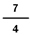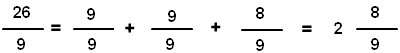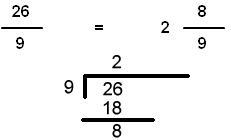# Fraction to mixed with circle models

## Learn or teach by designing your own fraction(improper) form to mixed form examples. All examples are modeled with circles.

FRACTION TO MIXED WITH CIRCLE MODELS
SHOW COLOR
EXPLAIN
SHOW INPUT
FRACTION FORM TO WHOLE OR MIXED FORM

# INSTRUCTIONS

With Fraction to Mixed with Circles Designer you can design fractions examples that use circle models to picture renaming fractions from fraction form to mixed form.

A mixed fraction is a fraction greater than one (1) that has a whole number part and a fraction number part, such as 1 34.

If you enter a fraction where the numerator is greater than or equal to the value of the denominator, such as, the program will show the mixed form of the number(1 34).

You can input any fraction with a value less than 5. The denominator must be less than 100. Press the <OK> button and the chosen fraction will appear in mixed form.

On the left is a <SHOW COLOR> check box. Uncheck the box to turn off the red selected parts of the circle. This will allow the learner to select the fraction.

Uncheck the <EXPLAIN> check box to turn off the answer and the explanation. You can ask your students to complete the number sentence.

With <SHOW INPUT> unchecked, the numerator and denominator input boxes will act the same as password input boxes so students will not see the numbers you input.

With <SHOW INPUT> unchecked and <EXPLAIN> unchecked, the learner can write a sentence that shows the fraction form and mixed or whole form.

With <SHOW COLOR> unchecked and <EXPLAIN> unchecked, the student can shade the indicated fraction and write in mixed or whole form.

Suggestions:

Besides looking at the number line, there are other methods can be used to arrive at the answer. One method is to see how many 99 there are in the fraction 269. In this case there are 2 units of 99 in 269, giving a whole number 2. After 2 units of 99 there are 8 more parts, giving a numerator of 8.Another method is to divide the numerator by the denominator. The denominator divides twice into the numerator with a remainder of 8, giving a whole number of 2, a numerator of 8, and a denominator of 9. This method is illustrated below.WINDOWS COMPUTERS

Windows users can select any part of the screen by right clicking and selecting "Take a screenshot". Adjust to fit mage you want. This copies the selection into Windows Clipboard™. The screen can then be pasted into Windows Paint™ or your favorite imaging program. Or you can select "Download" which will put the image into your files "Download" folder.